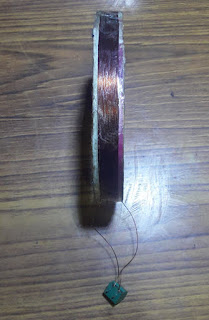# Colpitts Oscillator based search coil

A search coil can be used for metal detection and other application. Here it is shown how to build a search coil using Colpitts oscillator configuration. In colpitts oscillator, one inductor and two capacitors are used as the feedback circuit which determines the oscillation frequency. Here the search coil is used the inductor in the Colpitts oscillator.

The following shows the search coil.The search coil was made using AWG 26 enameled copper wire of 0.4mm diameter. To calculate the number of turns required for 126.65uH inductance wound around with 10.5cm diameter ring the online Air Core Inductance Online Calculator was used.

The calculated number of turns was 25.61 but later on experimenting 27 tight turns gave 100KHz. So 27 turns was used to make the search coil.

The inductance of the search coil was measured using PC based LCR meter and it was found that the coil had an inductance of 127uH.

The search coil inductance measurement setup is shown below.

The following shows Colpitts oscillator based search coil circuit.

The inductor L2 in the above circuit diagram represents the search coil. Although 126.65uH inductance was calculated for 100KHz oscillation frequency with C1 and C2 each of 40nF, upon measurement with oscilloscope the resonant frequency was 106.94KHz and so the inductance of the search coil is 110.75uH. To calculate the inductor value of 110uH the online Colpitts oscillator calculator was used.